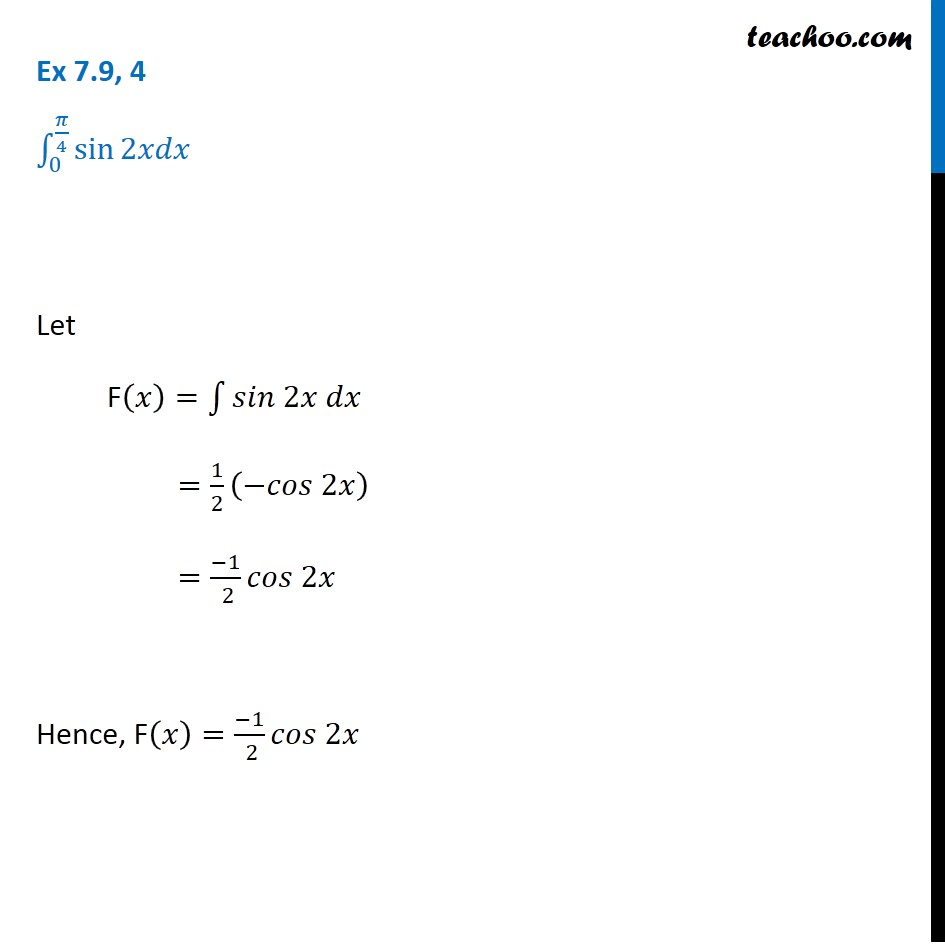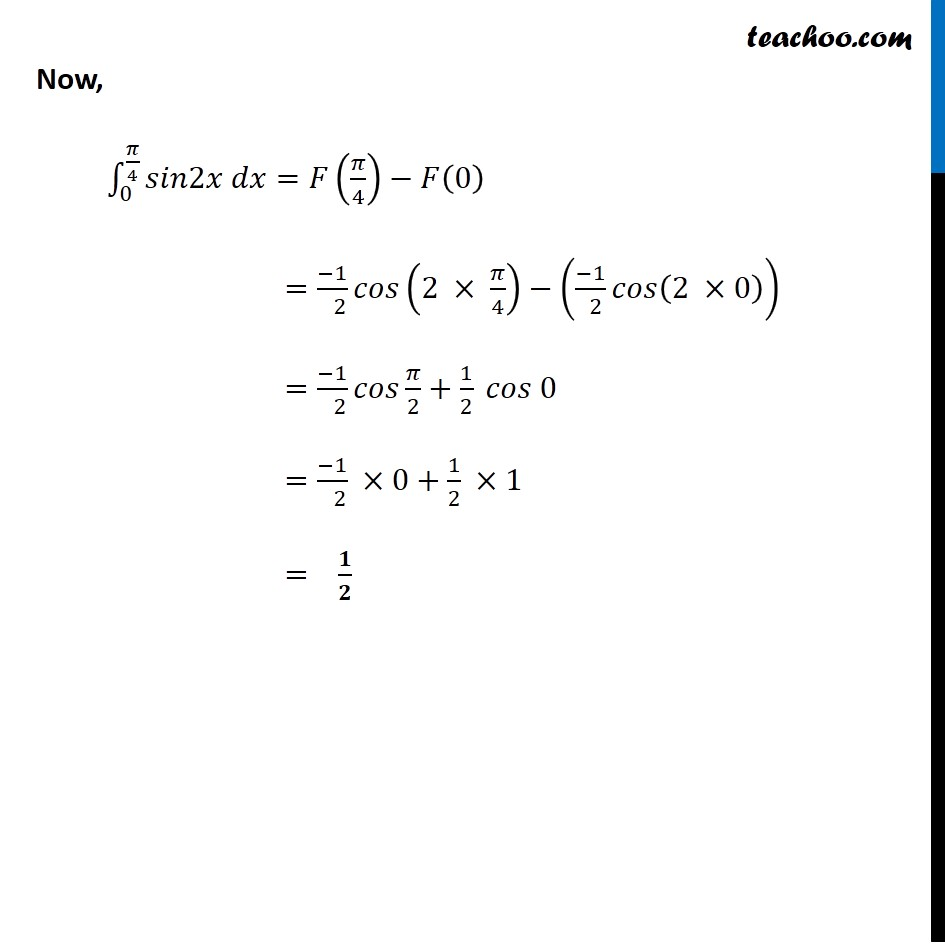Ex 7.8

Chapter 7 Class 12 Integrals
Serial order wiseLearn in your speed, with individual attention - Teachoo Maths 1-on-1 Class

### Transcript

Ex 7.8, 4 ∫_0^(𝜋/4)▒sin⁡2𝑥𝑑𝑥 Let F(𝑥)=∫1▒〖𝑠𝑖𝑛 2𝑥 𝑑𝑥〗 =1/2 (−𝑐𝑜𝑠 2𝑥) =(−1)/( 2) 𝑐𝑜𝑠 2𝑥 Hence, F(𝑥)=(−1)/( 2) 𝑐𝑜𝑠 2𝑥 Now, ∫_0^(𝜋/4)▒〖𝑠𝑖𝑛2𝑥 𝑑𝑥〗=𝐹(𝜋/4)−𝐹(0) =(−1)/( 2) 𝑐𝑜𝑠(2 × 𝜋/4)−((−1)/( 2) 𝑐𝑜𝑠(2 ×0)) =(−1)/( 2) 𝑐𝑜𝑠 𝜋/2+1/2 𝑐𝑜𝑠 0 =(−1)/( 2) ×0+1/2 ×1 = 𝟏/𝟐